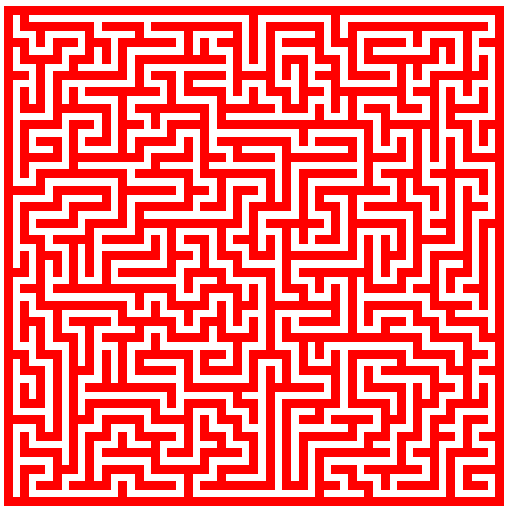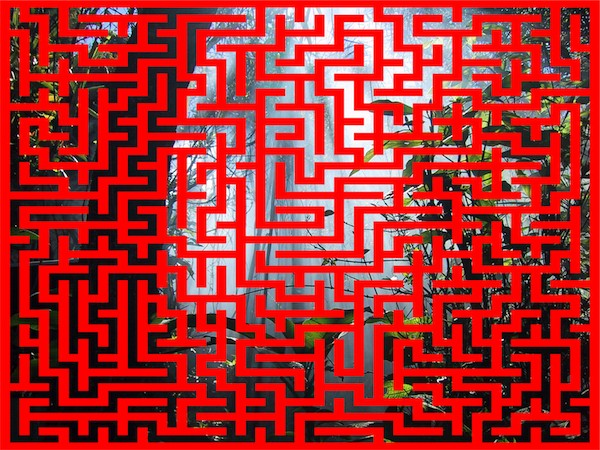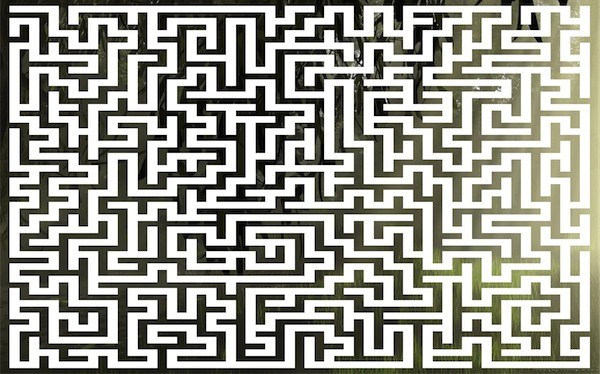Warning - this blog post has nothing worthwhile in it. No line of code in this post will be relevant, productive, mission critical, or "Web 2.0". It was as heck to play with though so if you are still interested, keep reading.

A few weeks back I blogged about how you could use ColdFusion to generate mazes. It worked ok, but I was wondering if I could do something a bit fun with it. For the first time in years I opened up my laptop while on my flight and - miracle of miracles - actually had enough room to type. The first thing I did was take my previous maze code and create a UDF out of it. This UDF took a size in width and height and returned a structure. The structure contained the array of maze data, as well as a new height and width (since the tile based maze is bigger than your original size). Here is the UDF:

`function genMaze(mazewidth, mazeheight) { var maze = arrayNew(2); var moves = []; var width = 2*mazeWidth+1; var height = 2*mazeHeight+1; var x = ""; var xPos = 2; var yPos = 2; var y = ""; var possibleDirections = ""; var move = ""; var back = ""; var s = {};for(x=1;x<=height;x++) { for(y=1;y<=width;y++){ maze[x][y]=1; } }maze[xPos][yPos]=0; moves = yPos+(xPos*width);while(arrayLen(moves)) {possibleDirections = "";if(arrayLen(maze) gte xPos+2 && maze[xPos+2][yPos] == 1 && xPos+2!=0 && xPos+2!=height-0){ possibleDirections &= "S"; }if(xpos-2 gt 0 && maze[xPos-2][yPos]==1 && xPos-2!=0 && xPos-2!=height-0){ possibleDirections &= "N"; } if(ypos-2 gt 0 && maze[xPos][yPos-2]==1 && yPos-2!=0 && yPos-2!=width-0){ possibleDirections &= "W"; } if(arrayLen(maze[xPos]) gte yPos+2 && maze[xPos][yPos+2]==1 && yPos+2!=0 && yPos+2!=width-0) { possibleDirections &= "E"; }if(len(possibleDirections)) { move = randRange(1,len(possibleDirections)); switch (mid(possibleDirections, move, 1)){case "N": maze[xPos-2][yPos]=0; maze[xPos-1][yPos]=0; xPos -=2; break; case "S": maze[xPos+2][yPos]=0; maze[xPos+1][yPos]=0; xPos +=2; break; case "W": maze[xPos][yPos-2]=0; maze[xPos][yPos-1]=0; yPos -=2; break; case "E": maze[xPos][yPos+2]=0; maze[xPos][yPos+1]=0; yPos +=2; break;} moves[arrayLen(moves)+1] = yPos + (xPos*width); } else { back = moves[arrayLen(moves)]; arrayDeleteAt(moves, arrayLen(moves)); xPos = fix(back/width); Ypos = back mod width; }}s.maze = maze; s.width = width; s.height = height; return s; }`

You can generate a maze by just doing:

`<cfset mazedata = genMaze(30,30)>`

So once I had that - I decided to see if I could create a graphical maze. I began by creating a canvas:

`<cfset canvas = imageNew("", 500, 500, "rgb", "white")>`

I then used a bit of math to determine the size of each 'block' (remember that mazedata is the result of the UDF call):

`<cfset sqWidth = canvas.width/mazedata.width> <cfset sqHeight = canvas.height/mazedata.height>`

Next, I set a drawing color:

`<!--- wall color ---> <cfset imageSetDrawingColor(canvas, "red")>`

and finally, here is the super complex code to draw the maze:

`<!--- begin to draw ---> <cfloop index="x" from="1" to="#mazedata.height#"> <cfloop index="y" from="1" to="#mazedata.width#"> <cfif mazedata.maze[x][y] is 1> <!--- draw a sq ---> <cfset yPos = (x-1) * sqHeight> <cfset xPos = (y-1) * sqWidth> <cfset imageDrawRect(canvas, xPos, yPos, sqWidth, sqHeight, true)> <cfelse> <!--- do nothing ---> </cfif> </cfloop> </cfloop><cfimage action="writeToBrowser" source="#canvas#">`

Remember that a 1 in our maze array means a wall. ColdFusion provides a rectangle drawing function for you, so the work is pretty trivial. And the result?Pretty cool I think. It's not super fast, but it only takes about one second. I was going to leave it at that, but then I decided - why not go crazy? Instead of a white canvas, what if we drew on a picture?Then - being the wild and crazy guy I am - I revered the draw logic. Instead of drawing walls, I drew the paths. (Note, this one has a different source image.)Pretty dumb - but it was cool to me. I've included a zip of the modified CFMs.